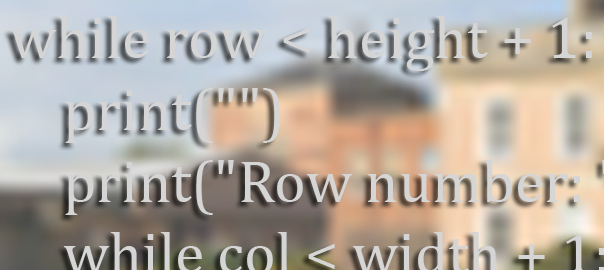# Extract RGB Values from an Image

Our Head of Physics today asked me if there was any code we could use that would demonstrate to his A-Level Physics students the way that digital images are represented by red, green and blue pixels with a value between 0 and 255. The reason is because of the introduction of a digital imaging unit within the new A-Level specification, and whilst this could be taught as pure theory he felt a working example to illustrate the point might help.

This got me thinking and after a bit of searching I found a Stack Overflow post which gave an example of retrieving a single pixel’s RGB value and printing it out.

This example makes use of the PIL python library, which unfortunately has not been updated for Python 3. However we can make use of the Pillow library in Python 3 to achieve the same thing!

The image I have used in the code is this one:First off you are going to need to install the Pillow library:

`pip3 install Pillow`

Below is my Python code which takes an input of an image (line 8). This image needs to be saved in the same directory as your Python script and I would suggest that it is not too big!

Click the “rgb-pixel.py” below to view the code.

```# Python script to display all pixels RGB values
# from an image and output them a row at a time
#
# Import the PIL library - pip3 install Pillow
from PIL import Image

# Open our image
im = Image.open("landscape.jpg")

# Convert our image to RGB
rgb_im = im.convert('RGB')

# Use the .size object to retrieve a tuple contain (width,height) of the image
# and assign them to width and height variables
width = rgb_im.size
height = rgb_im.size

# set some counters for current row and column and total pixels
row = 1
col = 1
pix = 0

# create an empty output row
rowdata = ""

# loop through each pixel in each row outputting RGB value as we go...
# all the plus and minus ones are to deal with the .getpixel class being
# zero indexed and we want the output to start at pixel 1,1 not 0,0!
while row < height + 1:
print("")
print("Row number: " + str(row))
while col < width + 1:
# get the RGB values from the current pixel
r, g, b = rgb_im.getpixel((col - 1, row - 1))
# append the RGB values to the rowdata variable as (R, G, B)
rowdata += "(" + str(r) + "," + str(g) + "," + str(b) + ") "
# increment the column count
col = col + 1
# increment the pixel count
pix = pix + 1
# print out all RGB values for the row
print(rowdata)
# clear out rowdata variable
rowdata = ""
# increment the row...
row = row + 1
# reset the column count
col = 1

# output for proof!
print("")
print("Width = " + str(width) + " pixels")
print("Height = " + str(height) + " pixels")
print("Total Pixels = " + str(pix) + ".")
```

I then thought it might be fun (albeit a complete waste of processing power!) to combine this with Python turtle and have the turtle redraw the image that we broke down into it’s RGB values!

On line 25 there is a variable called “sqsize”, this controls the size square the turtle draws for each pixel. If you set it to 1 then the turtle draw a straight line, however set it to anything above one and the turtle draws a square of that size for each pixel.

Be warned this is really inefficient code and will consume your CPU for no real purpose! Again, click the file name to see the code…

```# Import the PIL library - pip3 install Pillow
from PIL import Image
import turtle

# Open our image
im = Image.open("landscape.jpg")

# Convert our image to RGB
rgb_im = im.convert('RGB')

# Use the .size object to retrieve a tuple contain width,height of the image
# and assign them to width and height variables
width = rgb_im.size
height = rgb_im.size

# set some counters for current row and column and total pixels
row = 1
col = 1
pix = 0

# create an empty output row
rowdata = ""

# set up our turtle variables
sqsize = 2
turtle.colormode(255)
turtle.speed(0)

# loop through each pixel in each row outputting RGB value as we go...
while row < height + 1:
print("")
print("Row number: " + str(row))
while col < width + 1:
r, g, b = rgb_im.getpixel((col - 1, row - 1))
rowdata += "(" + str(r) + "," + str(g) + "," + str(b) + ") "
turtle.pendown()
turtle.fillcolor(r,g,b)
turtle.pencolor(r,g,b)
if sqsize > 1:
turtle.begin_fill()
for i in range(4):
turtle.forward(sqsize)
turtle.right(90)
turtle.end_fill()
else:
turtle.forward(sqsize)
turtle.penup()
if sqsize > 1:
turtle.forward(sqsize)
col = col + 1
pix = pix + 1
turtle.backward(sqsize * width)
turtle.right(90)
turtle.forward(sqsize)
turtle.left(90)
print(rowdata)
rowdata = ""
row = row + 1
col = 1

```

## 1 thought on “Extract RGB Values from an Image”

1.yudistira says: